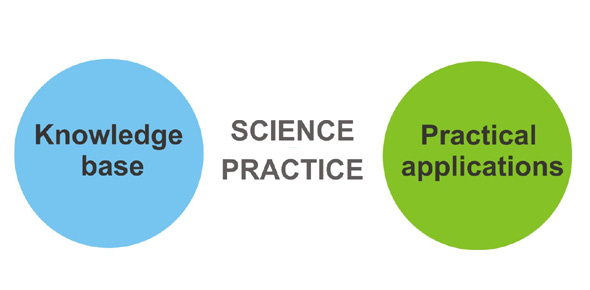# St. Gabe's Practice Science Test Chapter 2 - Observing States Of Matter

33 Questions | Attempts: 262
ShareSettingsThis is a practice test for science students who use St. Gabe's practice tests and are preparing for a test. It is specifically on Observing States of Matter. Try out this quiz and see how much you know.

• 1.
Does orange juice change shape when poured from a carton into a glass?
• A.

Yes

• B.

No

• 2.
__________ is matter that has no definite volume or shape.
• A.

Solids

• B.

Liquids

• C.

Gases

• D.

Bananas

• 3.
________ spreads out to fill its container.
• A.

Jello

• B.

Root Beer

• C.

Jelly

• D.

Gases

• E.

Oxygen

• 4.
Which of the following are some properties of water?
• A.

Boiling, freezing, room temperature

• B.

Hot, cold, warm

• C.

Liquid, solid, gas

• D.

Bottled, tap, pitcher

• E.

None of the above

• 5.
Scientists discovered much of what is known about matter by studying________
• A.

Bacteria

• B.

Greek history

• C.

Gases

• D.

Astronomy

• 6.
Early scientists concluded that all matter is made of ________ or _____________.
• A.

Pathogens or properties

• B.

Gold or silver

• C.

Tiny bits or particles

• D.

Oxygen or nuclei

• E.

None of the above

• 7.
True or False:  The particles that make up matter are very large and can be seen with a magnifying glass or microscope.
• A.

True

• B.

False

• 8.
An __________ is any material made up of only one kind of matter.
• A.

Atom

• B.

Nucleus

• C.

Element

• D.

Particle

• 9.
An atom is ______________-
• A.

The particles that are packed closely together

• B.

The director of the gases, neutrons, and electrons that move

• C.

The smalles part of an element that has the properties of that element

• D.

None of the above

• 10.
True or False:  All of the atoms in a given element are the same.
• A.

True

• B.

False

• 11.
When scientists study matter and its changes, they record their ______________.
• 12.
To save time and space, scientist use __________.
• 13.
A _________ _____________ is one or two letters that stand for the name of an element.
• A.

Greek letter

• B.

Roman numeral

• C.

Chemical symbol

• D.

Scientific legend

• E.

Scientific shortcut

• 14.
What is the symbol for the element oxygen?
• A.

Na

• B.

Fe

• C.

Au

• D.

O

• E.

O

• 15.
What is the symbol for the element iodine?
• A.

O

• B.

Fe

• C.

I

• D.

He

• E.

Io

• 16.
What element is represented by the following symbol:  "He"
• A.

Hydrogen

• B.

Iron

• C.

Helium

• D.

He-man

• 17.
What element is represented by the symbol "H"
• A.

Helium

• B.

Hypnon

• C.

Hydrogen

• D.

Hexapater

• 18.
True or False:  Particles in a liquid are farther apart and move faster than those in a solid. They can move past each other, allowing the liquid to take the shape of its container.
• A.

True

• B.

False

• 19.
True or False:  Particles in gases are close together and move slower than particles in solids or liquids. As the particles move, they remain clustered together and take up a small amount of space in their container.
• A.

True

• B.

False

• 20.
Some elements - particularly metals such as gold - are said to be _______________.
• 21.
The main reason that gold is valuable is because it is ____________.
• A.

Common

• B.

Needed to generate heat

• C.

Rare

• D.

• 22.
A ________ is a kind of matter made up of two or more elements that are joined together.
• A.

Element

• B.

Mixture

• C.

Solution

• D.

Compound

• 23.
_________ and compounds make up a large class of matter that is known as substances.
• 24.
The common compound salt is made of two elements: _________ and ____.
• A.

Carbon dioxide and oxygen

• B.

Sodium and chlorine

• C.

Hydrogen and iron

• 25.
A _______________ is a group of symbols that show the kind and number of each element in a single unit of a compound.
• A.

Molecular strategy

• B.

Chemical formula

• C.

Chemical array

• D.

Chemical VEN

## Related TopicsBack to top
×

Wait!
Here's an interesting quiz for you.